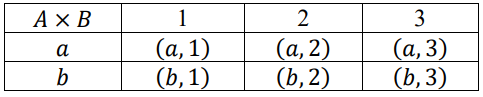# MA.912.LT.5.4Export Print
Perform the set operations of taking the complement of a set and the union, intersection, difference and product of two sets.

### Clarifications

Clarification 1: Instruction includes the connection to probability and the words AND, OR and NOT.
General Information
Subject Area: Mathematics (B.E.S.T.)
Strand: Logic and Discrete Theory
Status: State Board Approved

## Benchmark Instructional Guide

### Vertical Alignment

Previous Benchmarks

Next Benchmarks

### Purpose and Instructional Strategies

In Math for College Liberal Arts students will learn to perform set operations including complement, union, intersection, difference and product of two sets. In later classes, students will continue working with these operations.
• Instruction includes connection to MA.912.LT.5.5 and using Venn Diagrams to help students visualize set operations.
• Instruction includes discussion of a universal set U which contains all the possible elements of a set.
• Instruction includes finding the complement of a set denoted by the word NOT. The complement of a set A is all elements in the universal set that are not in the set A. The complement is written A′. Other benchmarks may refer to the complement set as Ac ,-A-, A′ or ~A
• Example:
• Given U = {1,2,3,4,5,6,7} and A = {2,4,6}, A′ = {1,3,5,7}.
• Instruction includes finding the union of two sets denoted by the word OR. The union of sets A and B is the set of all elements in set A OR in set B OR in both sets. The union of sets A and B is written AB
• Example:
• Given A = {1,2,3,4,5} and B = {2,4,6,8}, A B = {1,2,3,4,5,6,8}.
• Instruction includes finding the intersection of two sets denoted by the word AND. The intersection of sets A and B is the set of all elements in both set A AND set B. The intersection of sets A and B is written A ∩ B
• Example:
• Given A = {1,2,3,4,5} and A = {2,4,6,8}, A ∩ B = {2,4}.
• Instruction includes finding the difference of two sets. The difference between sets A and B is the set of all elements in set A that are not in set B. The difference of sets A and B is written AB. Note that order is important when finding a difference.
• Example:
• Given A = {1,2,3,4,5} and B = {2,4,6,8}, AB = {1,3,5}.
• Example:
• Given A = {1,2,3,4,5} and B = {2,4,6,8}, B − A = {6,8}.
• Instruction includes finding the product of two sets. The product of sets A and B is the set of ordered pairs (a, b) where a is an element of set A and b is an element of set B. The product of sets is sometimes called the Cartesian product or cross product. The product of sets A and B is written A × B. Note that order is important when finding a product.
• Example:
• Given A = {a, b} and B = {1,2,3}, A × B = {(a, 1), (a, 2), (a, 3), (b, 1), (b, 2), (b, 3)}
• The product can be demonstrated in table form.• Example:
• Given A = {a, b} and B = {1,2,3}, B × A = {(1, a), (1, b), (2, a), (2, b), (3, a), (3, b)}
• Instruction makes the connection between set operations and probability.

### Common Misconceptions or Errors

• Students may repeat elements that are in both sets when writing the union.
• Students may confuse union and intersection.
• Students may incorrectly apply the word “and” when applying set operations.

• Given U is the set of letters of the alphabet, A is the set of vowels and B is the set of letters that come before k, describe the following in words and then write the resulting sets in roster form:
a. A′
b. B′
c. A ∪ B
d. A ∩ B
e. AB
f. B − A
• Given set A is the set of even numbers less than or equal to six and set B is the set of odd numbers greater than 4 and less than 8, write the following sets in roster form.
a. A
b. B
c. A × B
d. B × A
e. A − B
f. B − A

### Instructional Items

Instructional Item 1
• Given A = {1,3,5,7,9} and B = {1,2,3,4,5}, find A ∩ B.
Instructional Item 1

• Given the sets:
A = {2, 5, 8, 9, 11, 13}
= {1, 2, 3, 4, 5, 9, 10}
• Find the following:
• A U B
• A ∩ B
• A ∩ B
*The strategies, tasks and items included in the B1G-M are examples and should not be considered comprehensive.

## Related Courses

This benchmark is part of these courses.
7912070: Access Mathematics for Liberal Arts (Specifically in versions: 2014 - 2015, 2015 - 2018, 2018 - 2019, 2019 - 2022, 2022 - 2023, 2023 and beyond (current))
1210305: Mathematics for College Statistics (Specifically in versions: 2022 and beyond (current))
1207350: Mathematics for College Liberal Arts (Specifically in versions: 2022 and beyond (current))
1212300: Discrete Mathematics Honors (Specifically in versions: 2022 and beyond (current))

## Related Access Points

Alternate version of this benchmark for students with significant cognitive disabilities.

## Related Resources

Vetted resources educators can use to teach the concepts and skills in this benchmark.

## Student Resources

Vetted resources students can use to learn the concepts and skills in this benchmark.

## Parent Resources

Vetted resources caregivers can use to help students learn the concepts and skills in this benchmark.